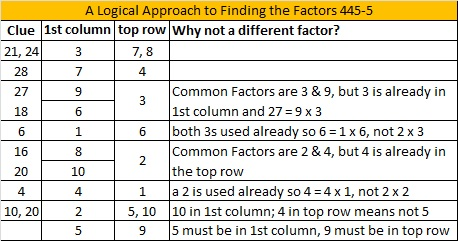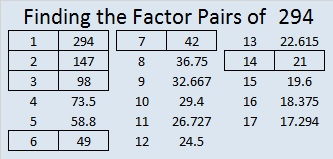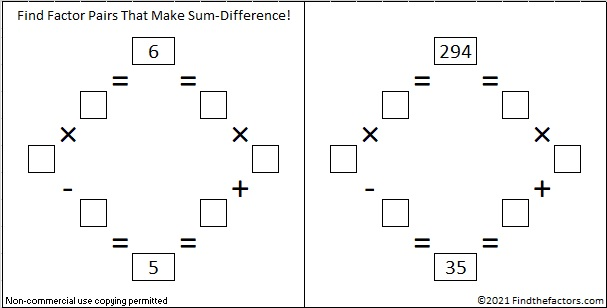# 294 and Level 5

### Today’s Puzzle:

Can you fill out this multiplication table that has only eleven clues?Print the puzzles or type the factors on this excel file: 10 Factors 2014-11-10### Factors of 294:

• 294 is a composite number.
• Prime factorization: 294 = 2 x 3 x 7 x 7, which can be written 294 = 2 x 3 x (7^2)
• The exponents in the prime factorization are 1, 1 and 2. Adding one to each and multiplying we get (1 + 1)(1 + 1)(2 + 1) = 2 x 2 x 3 = 12. Therefore 294 has exactly 12 factors.
• Factors of 294: 1, 2, 3, 6, 7, 14, 21, 42, 49, 98, 147, 294
• Factor pairs: 294 = 1 x 294, 2 x 147, 3 x 98, 6 x 49, 7 x 42, or 14 x 21
• Taking the factor pair with the largest square number factor, we get √294 = (√6)(√49) = 7√6 ≈ 17.146### Sum-Difference Puzzles:

6 has two factor pairs. One of them adds up to 5, and the other one subtracts to 5. Put those factor pairs in the correct boxes to solve the first puzzle.

294 has six factor pairs. Of of those factor pairs adds up to 35, and a different one subtracts to 35. Identify those factor pairs, and you will be able to solve the second puzzle.The second puzzle is really just the first puzzle in disguise.